How Cheenta works to ensure student success?
Explore the Back-Story

# Right-angled Triangle | AMC 10A, 2018 | Problem No 16Try this beautiful Problem on Geometry based on Right-angled triangle from AMC 10 A, 2018. You may use sequential hints to solve the problem.

## Right-angled triangle - AMC-10A, 2018- Problem 16

Right triangle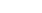has leg lengths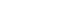and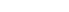. Including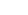and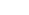, how many line segments with integer length can be drawn from vertex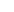to a point on hypotenuse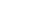,

•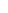•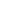•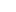•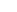•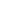Geometry

Triangle

Pythagoras

## Suggested Book | Source | Answer

Pre College Mathematics

#### Source of the problem

AMC-10A, 2018 Problem-16

#### Check the answer here, but try the problem first## Try with Hints

#### First Hint

Given that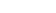is a Right-angle triangle andand. we have to find out how many line segments with integer length can be drawn from vertexto a point on hypotenuse?

Letbe the foot of the altitude fromto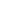. therefore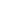is the shortest legth .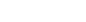which is betweenand.

Now can you finish the problem?

#### Second Hint

let us assume a line segmentwithonwhich is starts from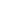to. So if we move this line segment the length will be decreases and the values will be look like as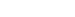. similarly if we moving this line segmentfromtohits all the integer values from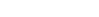.

Now Can you finish the Problem?

#### Third Hint

Therefore numbers of total line segments will be## Subscribe to Cheenta at Youtube

Try this beautiful Problem on Geometry based on Right-angled triangle from AMC 10 A, 2018. You may use sequential hints to solve the problem.

## Right-angled triangle - AMC-10A, 2018- Problem 16

Right trianglehas leg lengthsand. Includingand, how many line segments with integer length can be drawn from vertexto a point on hypotenuse,

•••••Geometry

Triangle

Pythagoras

## Suggested Book | Source | Answer

Pre College Mathematics

#### Source of the problem

AMC-10A, 2018 Problem-16

#### Check the answer here, but try the problem first## Try with Hints

#### First Hint

Given thatis a Right-angle triangle andand. we have to find out how many line segments with integer length can be drawn from vertexto a point on hypotenuse?

Letbe the foot of the altitude fromto. thereforeis the shortest legth .which is betweenand.

Now can you finish the problem?

#### Second Hint

let us assume a line segmentwithonwhich is starts fromto. So if we move this line segment the length will be decreases and the values will be look like as. similarly if we moving this line segmentfromtohits all the integer values from.

Now Can you finish the Problem?

#### Third Hint

Therefore numbers of total line segments will be## Subscribe to Cheenta at Youtube

This site uses Akismet to reduce spam. Learn how your comment data is processed.

### Knowledge Partner